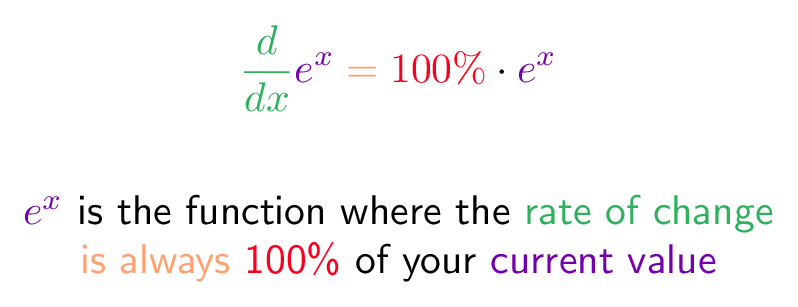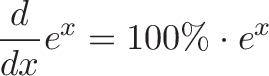# E (Derivative Definition)

## Colorized Definition\newcommand{\growth}{\color{c1}}
\newcommand{\change}{\color{c2}}
\newcommand{\unitInterest}{\color{c3}}
\newcommand{\currentAmount}{\color{c4}}
\newcommand{\equals}{\color{c5}}$\displaystyle{ \change \frac{d}{dx} \growth e^x \equals = \unitInterest {100\%} \plain \cdot \growth e^x }$

\growth     $e^x$
\plain      is the function where the
\change     rate of change
\
\equals      is always
\unitInterest    100\%
\plain         of your
\growth current value

## Read More - [An Intuitive Guide To Exponential Functions & e](https://betterexplained.com/articles/an-intuitive-guide-to-exponential-functions-e/) - [Common Definitions of e (Colorized)](https://betterexplained.com/articles/definitions-of-e-colorized/)